# 一、继承概述

1.为什么需要继承

c++最重要的特征是代码重用，通过继承机制可以利用已有的数据类型来定义新的数据类型，新的类不仅拥有旧类的成员，还拥有新定义的成员。

1. 一类是从基类继承过来的，一类是自己增加的成员。
2. 从基类继承过过来的表现其共性，而新增的成员体现了其个性。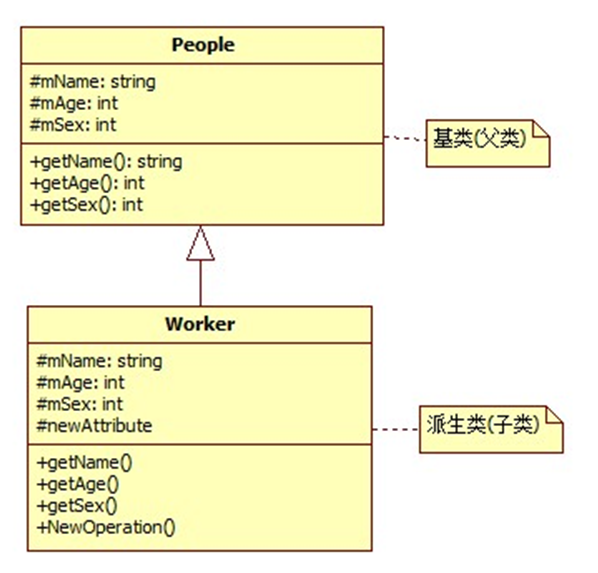2.派生类定义

Class 派生类名 :  继承方式 基类名{
//派生类新增的数据成员和成员函数
}

•   public ：    公有继承
•   private ：   私有继承
•   protected ： 保护继承

•   单继承：指每个派生类只直接继承了一个基类的特征
•   多继承：指多个基类派生出一个派生类的继承关系,多继承的派生类直接继承了不止一个基类的特征

# 二、派生类访问控制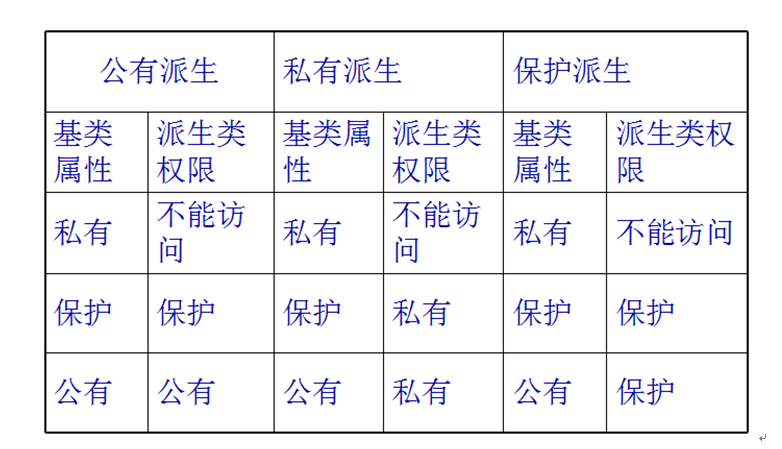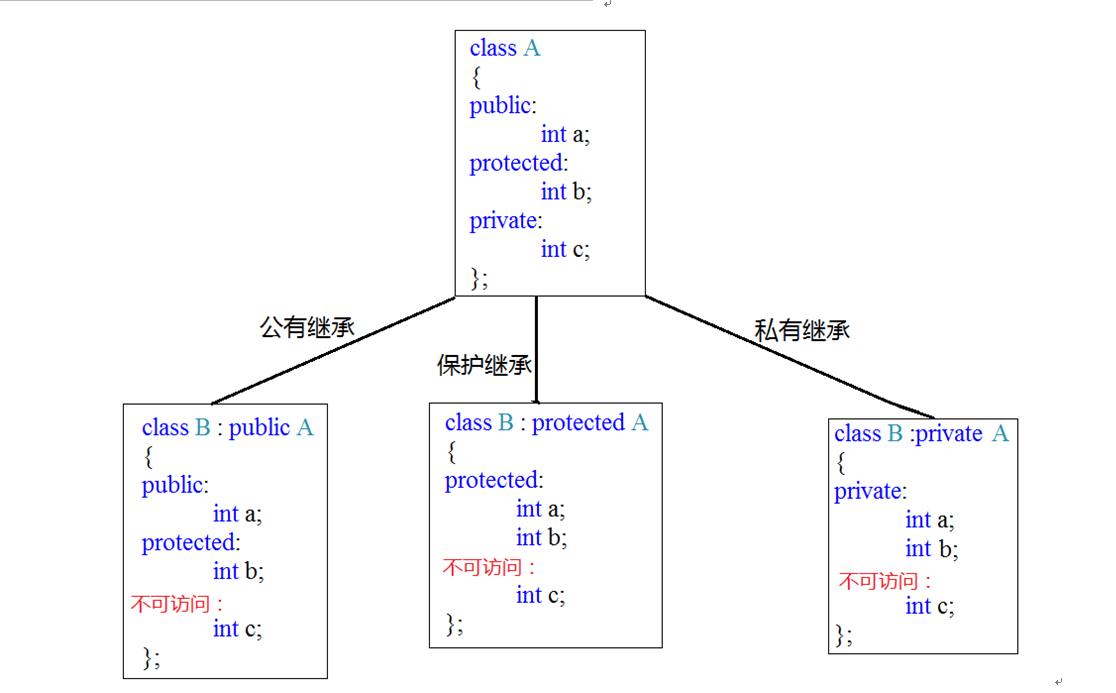#include <iostream>

using namespace std;

//基类
class A{
public:
int mA;
protected:
int mB;
private:
int mC;
};

//1. 公有(public)继承
class B : public A{
public:
void PrintB(){
cout << mA << endl; //可访问基类public属性
cout << mB << endl; //可访问基类protected属性
//cout << mC << endl; //不可访问基类private属性
}
};
class SubB : public B{
void PrintSubB(){
cout << mA << endl; //可访问基类public属性
cout << mB << endl; //可访问基类protected属性
//cout << mC << endl; //不可访问基类private属性
}
};
void test01(){

B b;
cout << b.mA << endl; //可访问基类public属性
//cout << b.mB << endl; //不可访问基类protected属性
//cout << b.mC << endl; //不可访问基类private属性
}

//2. 私有(private)继承
class C : private A{
public:
void PrintC(){
cout << mA << endl; //可访问基类public属性
cout << mB << endl; //可访问基类protected属性
//cout << mC << endl; //不可访问基类private属性
}
};
class SubC : public C{
void PrintSubC(){
//cout << mA << endl; //不可访问基类public属性
//cout << mB << endl; //不可访问基类protected属性
//cout << mC << endl; //不可访问基类private属性
}
};
void test02(){
C c;
//cout << c.mA << endl; //不可访问基类public属性
//cout << c.mB << endl; //不可访问基类protected属性
//cout << c.mC << endl; //不可访问基类private属性
}
//3. 保护(protected)继承
class D : protected A{
public:
void PrintD(){
cout << mA << endl; //可访问基类public属性
cout << mB << endl; //可访问基类protected属性
//cout << mC << endl; //不可访问基类private属性
}
};
class SubD : public D{
void PrintD(){
cout << mA << endl; //可访问基类public属性
cout << mB << endl; //可访问基类protected属性
//cout << mC << endl; //不可访问基类private属性
}
};
void test03(){
D d;
//cout << d.mA << endl; //不可访问基类public属性
//cout << d.mB << endl; //不可访问基类protected属性
//cout << d.mC << endl; //不可访问基类private属性
}

int main() {

B b;
SubB subB;
SubC subC;
D d;
SubD subD;

b.PrintB();
subB.PrintB();
subC.PrintC();
d.PrintD();
//subD.PrintD();

test01();
test02();
test03();

return EXIT_SUCCESS;
}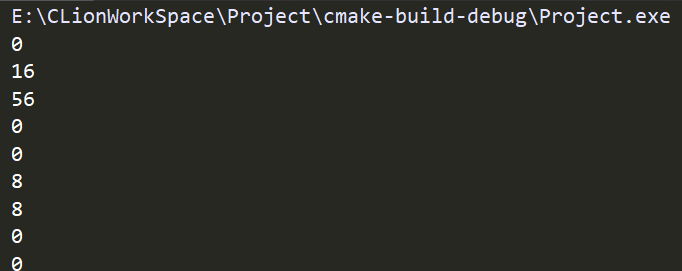# 三、继承中的构造和析构

1.继承中的对象模型

#include <iostream>

using namespace std;

class Aclass{
public:
int mA;
int mB;
};
class Bclass : public Aclass{
public:
int mC;
};
class Cclass : public Bclass{
public:
int mD;
};

void test(){
cout << "A size:" << sizeof(Aclass) << endl;
cout << "B size:" << sizeof(Bclass) << endl;
cout << "C size:" << sizeof(Cclass) << endl;
}

int main() {

test();

return EXIT_SUCCESS;
}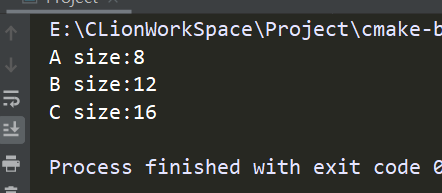2.对象构造和析构的调用原则

• 子类对象在创建时会首先调用父类的构造函数
• 父类构造函数执行完毕后，才会调用子类的构造函数
• 当父类构造函数有参数时，需要在子类初始化列表(参数列表)中显示调用父类构造函数
• 析构函数调用顺序和构造函数相反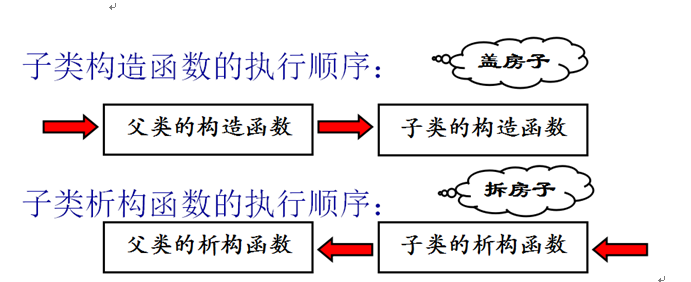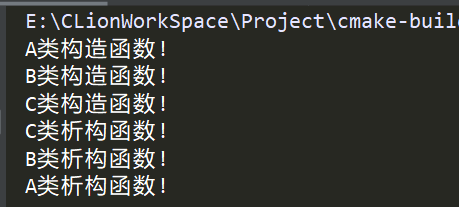# 四、多继承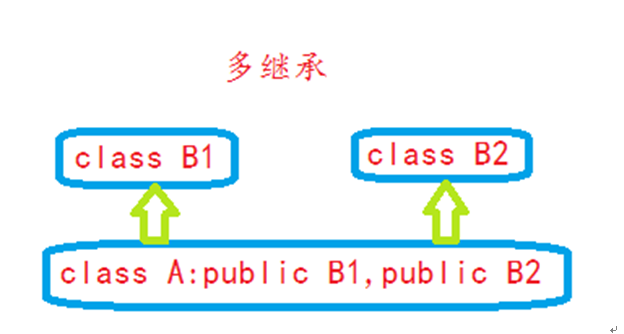class Base1{
public:
void func1(){ cout << "Base1::func1" << endl; }
};
class Base2{
public:
void func1(){ cout << "Base2::func1" << endl; }
void func2(){ cout << "Base2::func2" << endl; }
};
//派生类继承Base1、Base2
class Derived : public Base1, public Base2{};
int main(){

Derived derived;
//func1是从Base1继承来的还是从Base2继承来的？
//derived.func1();
derived.func2();

//解决歧义:显示指定调用那个基类的func1
derived.Base1::func1();
derived.Base2::func1();

return EXIT_SUCCESS;
}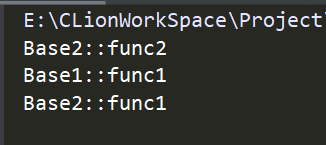posted @ 2019-10-13 19:12  rnaNg0丶  阅读(896)  评论(0编辑  收藏  举报